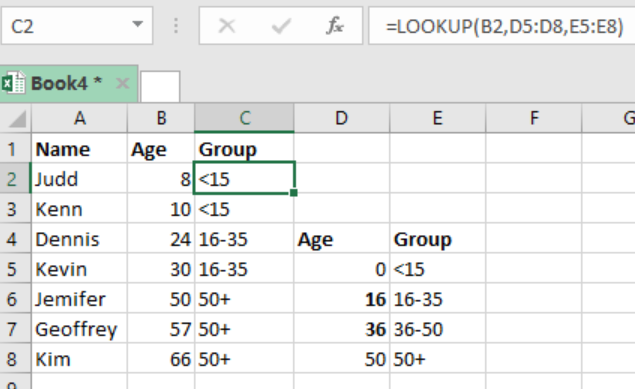Get instant live expert help with Excel or Google Sheets“My Excelchat expert helped me in less than 20 minutes, saving me what would have been 5 hours of work!”

#### Post your problem and you’ll get Expert help in seconds.

Your message must be at least 40 characters
Our professional Expert are available now. Your privacy is guaranteed.

# How to Group Numbers at Uneven Intervals in Excel

There are times when we are required to group numbers into intervals of unequal sizes. To do this, we need to understand how to use the LOOKUP function in excel. This article provides a clear guide on how to group numbers at uneven intervals in excel.Figure 1: How to group numbers at uneven intervals

## General syntax of the formula

`=LOOKUP(value, intervals, groups)`

## Explanation of the formula

The LOOKUP function plays a fundamental role in helping us group at uneven intervals. In the example above, the groups are in column C.

The formula in cells C2 is `=LOOKUP(B2,D5:D8,E5:E8)`

## Understanding how the formula works

• The function is configured in a way that allows it to lookup values in the ranges supplied. In our example above, the function is configured as follows;
• The lookup values are ages found in column B.
• The lookup vector is the named range “age”, D5:D8
• Result vector is names as “group” which is E5:E8
• The above setup allows the LOOKUP function to perform an appropriate match in the numeric values in the column D. it then returns the values associated to it from column E.

## Things to note

• LOOKUP function will return a corresponding group is it finds an exact match in the age column,
• In the event that the function does not find an exact match, it will traverse the age column until it finds a larger value.
• The function will return the largest value which is associated with the 50+
• The LOOKUP function returns an #N/A error in the event age is less than the smallest value in the age column.
• For this function to work appropriately, the ages must appear in an ascending order.

## Instant Connection to an Expert through our Excelchat Service

Most of the time, the problem you will need to solve will be more complex than a simple application of a formula or function. If you want to save hours of research and frustration, try our live Excelchat service! Our Excel Experts are available 24/7 to answer any Excel question you may have. We guarantee a connection within 30 seconds and a customized solution within 20 minutes.

### Did this post not answer your question? Get a solution from connecting with the expert.Another blog reader asked this question today on Excelchat:## Subscribe to Excelchat.coAnother blog reader asked this question today on Excelchat: